• 1、 理解自底向上语法分析方法； 2、 用LR分析技术实现语法分析器； 3、 熟练掌握LR分析程序的构造方法。
• 1） 任意输入一个文法G； 2） 判断该文法是否为算符文法；... 对应的语法树；能够输出分析过程中每一步符号 栈的变化情况以及根据当前最左素短语进行归约 的过程。如果该句子非法则进行相应的报错处理。
•编译原理
• 从分析树的底部(叶节点向顶部根节点方向构造分析...可以看成是将输入串归约为文法开始符号S的过程【顶向下的语法分析采用最左推导方式自底向上语法分析采用最左归约方式(反向构造最右推导)】 每次规约“句柄” ...

参考哈工大课件

自底向上的语法分析

从分析树的底部(叶节点向顶部根节点方向构造分析树，可以看成是将输入串归约为文法开始符号S的过程

自顶向下的语法分析采用最左推导方式；
自底向上的语法分析采用最左归约方式，实际上是 反向构造最右推导

自底向上语法分析的通用框架：移入-归约分析

1）在对输入串的一次从左到右扫描过程中，语法分析器将零个或多个输入符号移入到栈的顶端，直到它可以对栈顶的一个文法符号串β进行归约为止
2）它将β归约为某个产生式的左部
3）语法分析器不断地重复这个循环，直到它检测到一个语法错误，或者栈中包含了开始符号且输入缓冲区为空(当进入这样的格局时，语法分析器停止运行，并宣称成功完成了语法分析)为止
注意：每步规约都是规范规约，每次规约“句柄”
移入-归约分析器可采取的4种动作：

• 移入：将下一个输入符号移到栈的顶端
• 归约：被归约的符号串的右端必然处于栈顶。语法分析器在栈中确定这个串的左端，并决定用哪个非终结符来替换这个串
• 接收：宣布语法分析过程成功完成
• 报错：发现一个语法错误，并调用错误恢复子例程

移入-归约分析中存在的问题
造成错误的原因：错误地识别了句柄

LR分析法概述

LR文法是最大的、可以构造出相应移入-归约语法分析器的文法类

L: 对输入进行从左到右的扫描
R: 反向构造出一个最右推导序列
LR(k)分析：需要向前查看k个输入符号的LR分析。当省略(k)时，表示k =1

1. LR 分析法的基本原理
自底向上分析的关键问题是：如何正确地识别句柄。
句柄是逐步形成的，用“状态”表示句柄识别的进展程度
LR分析器基于这样一些状态来 构造自动机进行句柄的识别

例：S→bBB
S→·bBB 移进状态
S→b·BB / S→bB·B 移进、待归约状态
S→bBB· 归约状态
注意： 产生式A→ε 只生成一个项目A→ ·

1. LR 分析器（自动机）的总体结构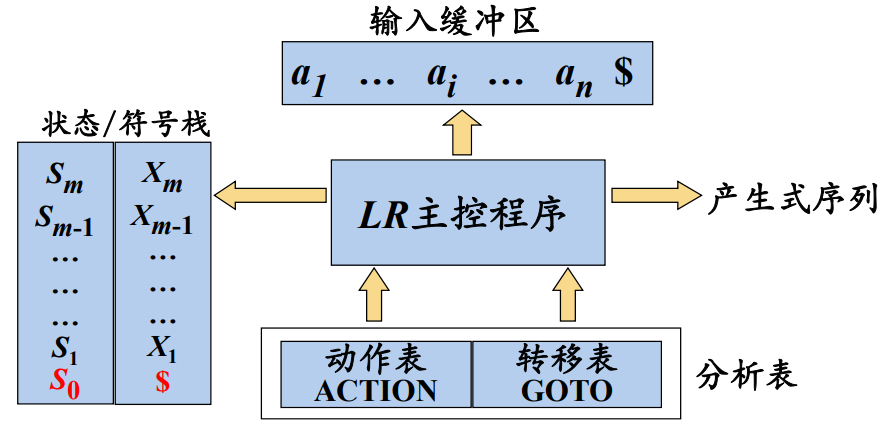LR 分析表的结构：
• sn：将符号a、状态n 压入栈
• rn：用第n个产生式进行归约

例：文法
① S→BB
② B→aB
③ B→b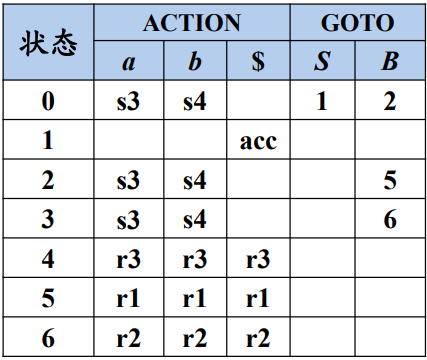输入bab#

状态剩余输入动作
0#bab#
04#bab#0和b比较s4，移入b，添加4
02#Bab#4和a比较r3，归约文法B→b右部长度1，在符号栈和状态栈中分别移出1个符号，在符号栈中进入B。此时栈顶是0，遇到刚规约出的B，到状态2，添加2状态
023#Bab#2和a比较s3，移入a，添加3
0234#Bab#3和b比较s4，移入b，添加4
0236#BaB#4和#r3，归约文法B→b，4和b出栈，B进栈。3和B比较到状态6，6进栈
025#BB#6和#比较r2，B→aB，36、aB出栈B进栈。2和B到到状态5，5入栈
0#S#5和#比较r1，S→BB，25、BB出栈，S入栈。
01#S#0和S比较到状态1，1入栈
1遇到#成功接收acc
• 如果ACTION[sm , ai]=acc，那么分析成功
• 如果ACTION[sm , ai]=err ，那么出现语法错误
//LR 分析算法
输入：串w和LR语法分析表，该表描述了文法G的ACTION函数和GOTO函数。
输出：如果w在 L(G)中，则输出w的自底向上语法分析过程中的归约步骤；否则给出一个错误指示。
方法：初始时，语法分析器栈中的内容为初始状态s0，输入缓冲区中的内容为w#。
令a为w$的第一个符号； while(1) { /* 永远重复*/ 令s是栈顶的状态； if ( ACTION [s，a] = st ) { 将t压入栈中； 令a为下一个输入符号； } else if (ACTION [s，a] =归约A → β ) { 从栈中弹出│ β │个符号； 将GOTO [t，A]压入栈中； 输出产生式 A → β ； } else if (ACTION [s，a] =接受) break/* 语法分析完成*/ else调用错误恢复例程； } LR(0)分析 增广文法：如果G 是一个以S为开始符号的文法，则G的增广文法 G’ 就是在G中加上新开始符号S’ 和产生式S’ → S而得到的文法 引入增广文法的目的：使新的开始产生式的目的是使得文法开始符号仅出现在一个产生式的左边，从而使得分析器只有一个接受状态 可以把等价的项目组成一个项目集( I ) ，称为项目集闭包(Closure of Item Sets)，每个项目集闭包对应着自动机的一个状态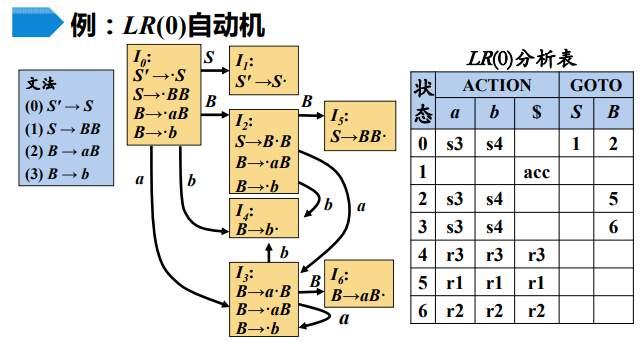CLOSURE()函数 计算给定项目集I的闭包： CLOSURE(I) = I∪{B→ · γ | A→α·Bβ∈CLOSURE(I) , B→γ∈P} //CLOSURE()函数 SetOfltems CLOSURE ( I ) { J = I； repeat for ( J中的每个项A → α·Bβ ) for ( G的每个产生式B → γ ) if ( 项B → · γ不在J中 ) 将B → · γ加入J中； until 在某一轮中没有新的项被加入到J中； return J； } LR(0) 分析过程中的冲突：移进/归约冲突& 归约归约冲突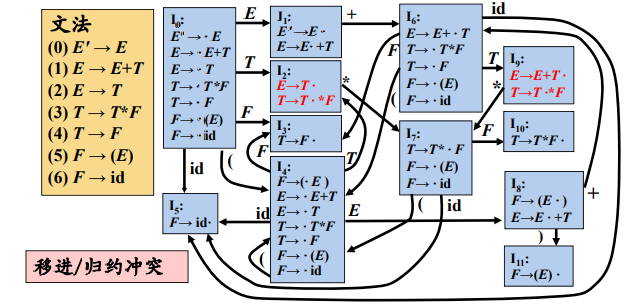表达式文法的LR(0)分析表含有移进/归约冲突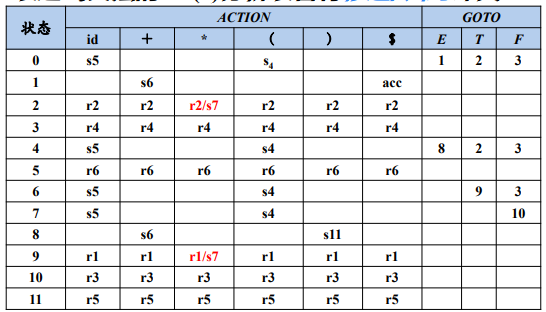如果LR(0)分析表中没有语法分析动作冲突，那么给定的文法就称为LR(0)文法 • 不是所有CFG都能用LR(0)方法进行分析，也就是说，CFG不总是LR(0)文法 SLR分析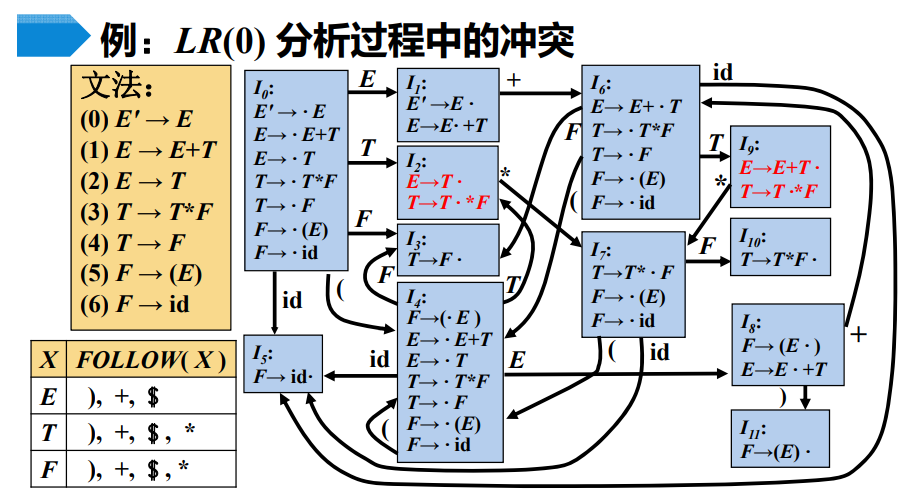在状态2，使用LR0文法，不向后看符号，即不考虑上下文。由followE可以知道如果下一个符号是*，则采取移进，则不会产生冲突。 SLR分析法的基本思想 如果集合{a1, a2, …, am}和FOLLOW(B1)， FOLLOW(B2)，…，FOLLOW(Bn)两两不相交，则项目集I中的冲突可以按以下原则解决： • 设a是下一个输入符号 若a∈{ a1, a2, …, am }，则移进a； 若a∈FOLLOW(Bi)，则用产生式Bi→γi 归约 此外，报错 由此就可以化解冲突，如果能够化解冲突则成为SLR文法。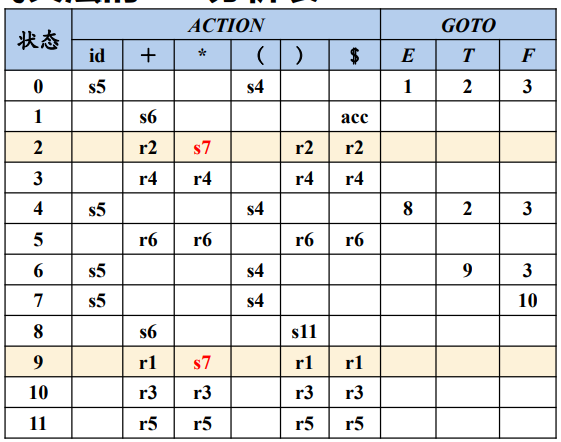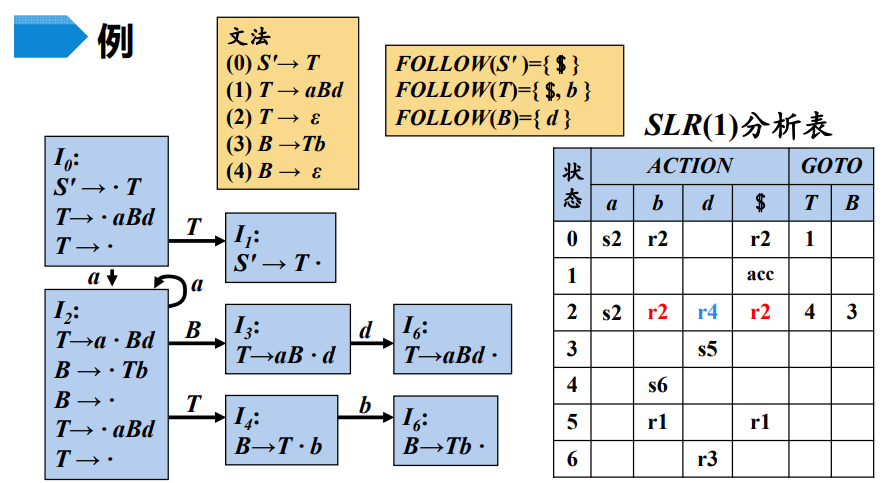只对于在follow集中的符号才进行归约 //SLR 分析表构造算法 构造G'的规范LR(0)项集族C = { I0, I1,, In}。 根据Ii构造得到状态i。状态i 的语法分析动作按照下面的方法决定： if A→α·aβ∈Ii and GOTO( Ii , a )=Ij then ACTION[ i, a ]=sj if A→α.Bβ∈Ii and GOTO( Ii , B )=Ij then GOTO[ i, B ]=j if A→α·∈Ii且A ≠ S' then for a∈FOLLOW(A) do ACTION[ i, a ]=rj （j是产生式A→α的编号） if S'→S·∈Ii then ACTION [ i ,$ ]=acc;
没有定义的所有条目都设置为“error”。

SLR分析存在的问题：
SLR只是简单地考察下一个输入符号b是否属于与归约项目A→α相关联的FOLLOW(A)，但b∈FOLLOW(A)
只是归约α的一个必要条件，而非充分条件

LR(1)分析

对于产生式 A→α的归约，在不同的使用位置，A会要求不同的后继符号。在特定位置，A的后继符集合是FOLLOW(A)的子集。
规范LR(1)项目：
将一般形式为 [A→α·β, a]的项称为 LR(1) 项，其中A→αβ 是一个产生式，a 是一个终结符(这里将\$视为一个特殊的终结符)

• LR(1) 中的1指的是项的第二个分量的长度
• 在形如[A→α·β, a]且β ≠ ε的项中，展望符a没有任何作用
• 但是一个形如[A→α·, a]的项在只有在下一个输入符号等于a时才可以按照A→α 进行归约。这样的a的集合总是FOLLOW(A)的子集，而且它通常是一个真子集。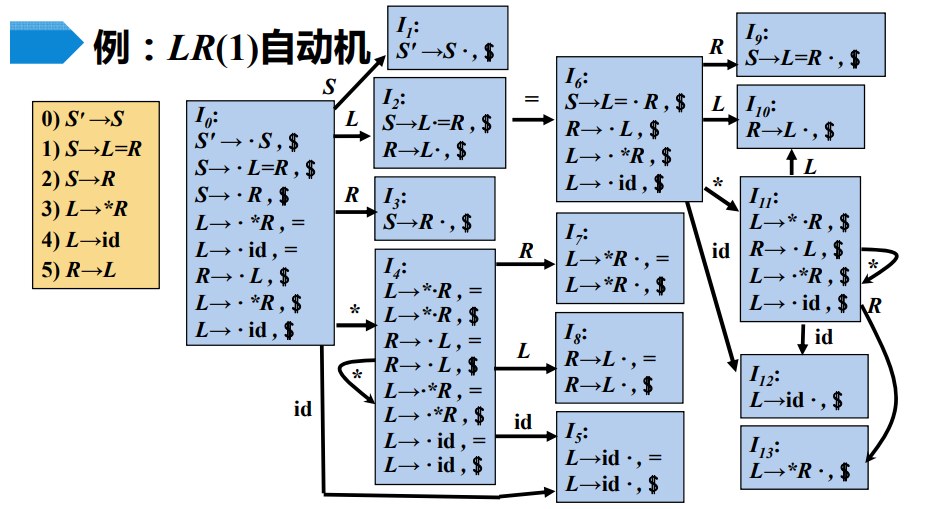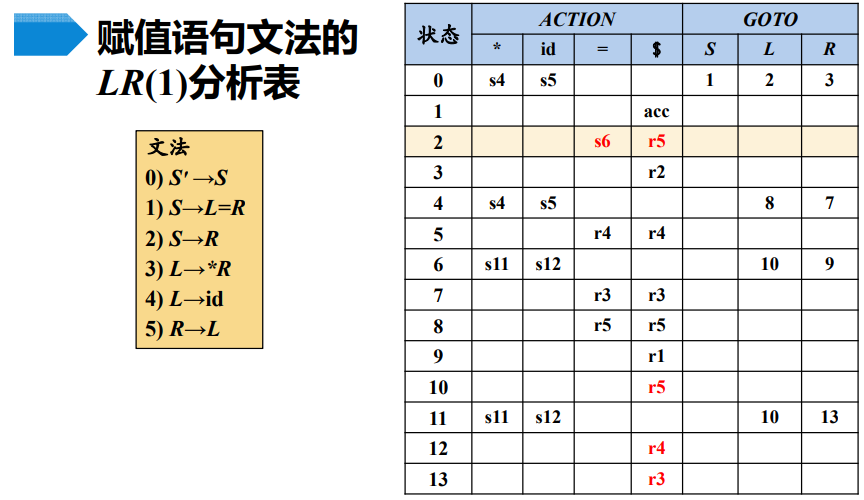展开全文• 实验三 自底向上的语法分析

千次阅读 2018-06-18 08:41:48
// 首先，感谢孙同学的Java版代码，让我有了参考 // 下面上C++代码，文件操作部分自行改写即可 #include &amp;lt;iostream&amp;gt; #include &amp;lt;fstream&amp;gt; ...amp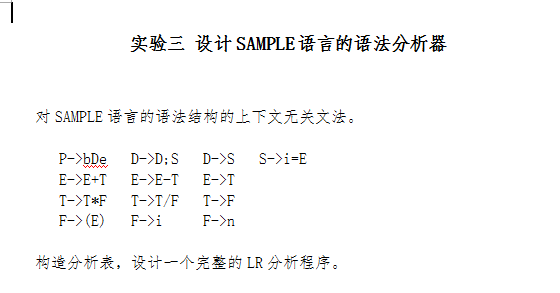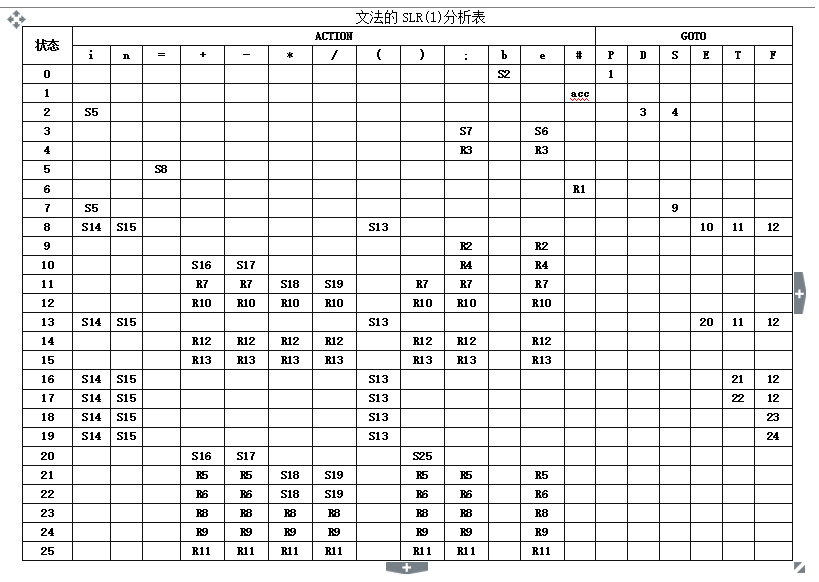// 首先，感谢孙同学的Java版代码，让我有了参考
// 下面上C++代码，文件操作部分自行改写即可
#include <iostream>
#include <fstream>
#include <cstring>
#include <stack>
using namespace std;

stack<string> state_st; // 状态栈
stack<string> sign_st; // 符号栈
string input_str = ""; // 输入串
string action_table[] = { {"0","b","S2"}, {"1","#","acc"}, {"2","i","S5"}, {"3",";","S7"},
{"3","e","S6"}, {"4",";","R3"}, {"4","e","R3"}, {"5","=","S8"},
{"6","#","R1"}, {"7","i","S5"}, {"8","i","S14"}, {"8","n","S15"},
{"8","(","S13"}, {"9",";","R2"}, {"9","e","R2"}, {"10","+","S16"},
{"10","-","S17"}, {"10",";","R4"}, {"10","e","R4"}, {"11","+","R7"},
{"11","-","R7"}, {"11","*","S18"}, {"11","/","S19"}, {"11",")","R7"},
{"11",";","R7"}, {"11","e","R7"}, {"12","+","R10"}, {"12","-","R10"},
{"12","*","R10"}, {"12","/","R10"}, {"12",")","R10"}, {"12",";","R10"},
{"12","e","R10"}, {"13","i","S14"}, {"13","n","S15"}, {"13","(","S13"},
{"14","+","R12"}, {"14","-","R12"}, {"14","*","R12"}, {"14","/","R12"},
{"14",")","R12"}, {"14",";","R12"}, {"14","e","R12"}, {"15","+","R13"},
{"15","-","R13"}, {"15","*","R13"}, {"15","/","R13"}, {"15",")","R13"},
{"15",";","R13"}, {"15","e","R13"}, {"16","i","S14"}, {"16","n","S15"},
{"16","(","S13"}, {"17","i","S14"}, {"17","n","S15"}, {"17","(","S13"},
{"18","i","S14"}, {"18","n","S15"}, {"18","(","S13"}, {"19","i","S14"},
{"19","n","S15"}, {"19","(","S13"}, {"20","+","S16"}, {"20","-","S17"},
{"20",")","S25"}, {"21","+","R5"}, {"21","-","R5"}, {"21","*","S18"},
{"21","/","S19"}, {"21",")","R5"}, {"21",";","R5"}, {"21","e","R5"},
{"22","+","R6"}, {"22","-","R6"}, {"22","*","S18"}, {"22","/","S19"},
{"22",")","R6"}, {"22",";","R6"}, {"22","e","R6"}, {"23","+","R8"},
{"23","-","R8"}, {"23","*","R8"}, {"23","/","R8"}, {"23",")","R8"},
{"23",";","R8"}, {"23","e","R8"}, {"24","+","R9"}, {"24","-","R9"},
{"24","*","R9"}, {"24","/","R9"}, {"24",")","R9"}, {"24",";","R9"},
{"24","e","R9"}, {"25","+","R11"}, {"25","-","R11"}, {"25","*","R11"},
{"25","/","R11"}, {"25",")","R11"}, {"25",";","R11"}, {"25","e","R11"}
};
const int action_length = 100;
string goto_table[] = { {"0","P","1"}, {"2","D","3"}, {"2","S","4"}, {"7","S","9"},
{"8","E","10"}, {"8","T","11"}, {"8","F","12"}, {"13","E","20"},
{"13","T","11"}, {"13","F","12"}, {"16","T","21"}, {"16","F","12"},
{"17","T","22"}, {"17","F","12"}, {"18","F","23"}, {"19","F","24"},
};
int goto_length = 16;
string production[] = { {}, {"P","bDe"}, {"D","D;S"}, {"D","S"}, {"S","i=E"},
{"E","E+T"}, {"E","E-T"}, {"E","T"}, {"T","T*F"}, {"T","T/F"},
{"T","F"}, {"F","(E)"}, {"F","i"}, {"F","n"}
};
char symbol_table = { '=','+','-','*','/','(',')',';' };
int state_value[action_length] = {0};

{
fstream f;
f.open(sf,ios::in);
if(!f)  { cout<<"源文件打开失败!"; exit(1); }

f>>input_str;
f.close();
}

void get_state_value()
{
for(int i = 0; i < action_length; i++)
{
string s = action_table[i];
s.replace(0,1,"");
if(s.length() == 2)
{
state_value[i] = (s-48)*10 + (s-48);
}
else
{
state_value[i] = s - 48;
}
}
}

string return_state(string a, string b)
{
string str = "";
char ch = b.at(0);
// 输入串为非终结符
if(ch>='A' && ch<='Z')
{
for(int i = 0; i < goto_length; i++)
{
if((goto_table[i].compare(a) == 0) && (goto_table[i].compare(b) == 0))
{
str = goto_table[i];
}
}
}
// 输入串为终结符
else
{
if((ch>='a' && ch<='z') || ch == symbol_table ||
ch == symbol_table || ch == symbol_table ||
ch == symbol_table || ch == symbol_table ||
ch == symbol_table || ch == symbol_table ||
ch == symbol_table)
{
for(int i = 0; i < action_length; i++)
{
if((action_table[i].compare(a) == 0) &&
(action_table[i].compare(b) == 0))
{
string s = action_table[i];
str = s.replace(0,1,"");
}
}
}
}
return str;
}

void analyse()
{
input_str += "#";
bool success_flag = false;
bool state_flag = false;
// 状态栈，符号栈，剩余输入串
string state_s = "", sign_s = "", rest_str = input_str;
string current_s = ""; // 当前状态
string start_str = input_str.substr(0,1); // 输入串首字符
int next = 1, num = 1;

state_st.push("0"); sign_st.push("#");
state_s += "0"; sign_s += "#";
cout<<num<<"\t"<<state_st.top()<<"\t\t"<<sign_st.top()<<"\t\t"<<input_str<<endl;

while(!success_flag)
{
current_s = state_st.top();
for(int i = 0; i < action_length; i++)
{
if((action_table[i].compare(current_s) == 0) &&
(action_table[i].compare(start_str) == 0))
{
state_flag = true;
if(action_table[i] == "acc")
{
success_flag = true;
cout<<"------------------------"<<endl;
cout<<"语法分析成功（自下而上）"<<endl;
return ;
}
// 移入项
if(action_table[i].at(0) == 'S')
{
state_st.push(return_state(current_s, start_str));
state_s += return_state(current_s, start_str);;
sign_st.push(start_str);
sign_s += start_str;

start_str = input_str.at(next);
// cout<<start_str<<endl;
rest_str = input_str.substr(next, input_str.length()-1);
next++; num++;
}
// 规约项
if(action_table[i].at(0) == 'R')
{
for(int j = 0; j < production[state_value[i]].length(); j++)
{
sign_s = sign_s.substr(0, sign_s.length()-1);
string top_s = state_st.top();
state_st.pop();
state_s = state_s.substr(0, state_s.length() - top_s.length());
}
sign_st.push(production[state_value[i]]);
sign_s += production[state_value[i]];
state_s += return_state(state_st.top(), production[state_value[i]]);
state_st.push(return_state(state_st.top(), production[state_value[i]]));
num++;
}
}
}
if(!state_flag)
cout<<"error"<<endl; // 未处理
cout<<num<<"\t"<<state_s<<"\t\t"<<sign_s<<"\t\t"<<rest_str<<endl;
}
}

int main()
{
get_state_value();
analyse();
return 0;
}

运行结果如下：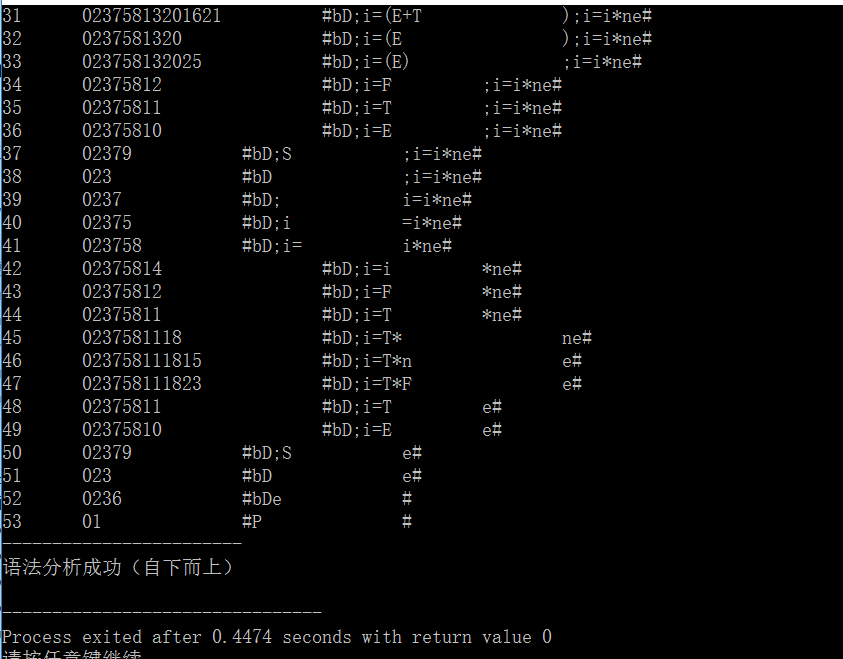展开全文自底向上 词法分析
• 自底向上分析语法分析程序设计与实现 一、实验任务及内容 编制一个文法的输入，从键盘、文件或文本框输入给定文法，依次存入输入缓冲区（字符型数据）。 编制一个算法，模拟LR（0）分析器的主控流程，实现对给定...

自底向上分析语法分析程序设计与实现

一、实验任务及内容

1. 编制一个文法的输入，从键盘、文件或文本框输入给定文法，依次存入输入缓冲区（字符型数据）。
2. 编制一个算法，模拟LR（0）分析器的主控流程，实现对给定文法的LR分析。最终生成LR（0）分析表。
3. 编制程序运行时间控制程序，并作为函数在主控程序调用。

二、实验环境

Windows系统、C++

三、实验的整个实现过程

(1) 本实验使用的数据结构主要有map、set、数组。
(2) 读入文件
首先读入文法文件，将其中的文法存放在productions中，并加入“S’->S”。接着利用productions构造圆点只放置于产生式右部开头的位置的拓广文法，之后构造新状态时会用到该拓广文法中的产生式。
(3) 构造项目集

• 构造I_0状态
使用产生式“S’->S”开始（本实验使用星号替代课本中的圆点），扫视能由*后的S能推出的产生式对应的项目，例如S->*A、S->*B。接下来分别以A、B等非终结符扫视，依次类推，直到I_0状态不再增加为止。
• 构造所有的项目集
以I_0为基本状态集，对于其中的每一个项目，如果圆点不是项目字符串最后的位置，则以圆点后面的字母作为输入符号，将其加入到新状态中，并将圆点向后移动。如果原点后是非终结符，则将该非终结符能推出的串加入到该新状态中。
如果此新状态与状态集中的某个状态相同，则删除记录。
在构造新状态时，记录各个状态之间的转换关系，存放到二维数组中，代表DFA图中的连线。
(4) 构造Action表与GoTo表根据DFA中记录的状态转换关系，可以很轻松的构造出Action表与GoTo表，使用二维数组存储。

四、完整代码

//!!!!!! 2020.11.26 QLU实验报告，QLUer勿抄袭!!!!!!
#include <iostream>
#include <time.h>
#include <fstream>
#include <set>
#include <map>
using namespace std;
ifstream input("wenfa.txt");
map<string, set<string> > productions;//读入文件后，存放产生式
map<string, set<string> > project;//在每个产生式右部前面加上点，得到初始项目集
map<int, set<string> > StateSet;//状态
map<int, set<string> > StateSetTemp;
string css_by_order;
string ActionTable;
int GoToTable;
set<string> Vn;
set<string> Vt;
string vn_index;
string vt_index;

bool isVn(char ch) {
if (ch >= 'A' && ch <= 'Z') {
return true;
} else{
return false;
}
}

//得到最初项目集
void getProject() {
productions["S\'"].insert("S");
//读入文法文件
int k = 1;
string temp;
while (getline(input, temp)) {
productions[temp.substr(0,1)].insert(temp.substr(3, temp.length() - 3 +1));
css_by_order[k++] = temp;
}
//构造基本项目集，即圆点位于右部开头的位置
string dot = "*";
for(map<string, set<string>>::iterator it = productions.begin(); it != productions.end(); it++) {
for(set<string>::iterator ij =it->second.begin(); ij != it->second.end(); ij++) {
string startStr = *ij;
startStr.insert(0, dot, 0, 1);
project[it->first].insert(startStr);
}
}
}

void Init_I0() {
StateSet.insert("S'->*S");
//先将所有的左部为S的产生式加入0号状态中
for(map<string, set<string> >::iterator it = project.begin(); it != project.end(); it++) {
if ((it->first) == "S") {
for(set<string>::iterator ij = it->second.begin(); ij != it->second.end(); ij++) {
StateSetTemp.insert(it->first + "->" + *ij);
}
}
}
//根据I0状态中已知内容，添加后续
while (! StateSetTemp.empty()){
set<string>::iterator it = StateSetTemp.begin();
string it_str = *it;//暂时集合中的每一条产生式
//如果点后面是非终结符，在project例找到它能推出来的产生式，加入temp,
if (isVn(it_str)) {
string after_dot_ch = it_str.substr(4,1);
for(set<string>::iterator css = project[after_dot_ch].begin(); css != project[after_dot_ch].end(); css++) {
StateSetTemp.insert(after_dot_ch + "->" + *css);
}
StateSetTemp.erase(it_str);
StateSet.insert(it_str);
}else if(! isVn(it_str)) {
StateSetTemp.erase(it_str);
StateSet.insert(it_str);
}
}
}

string MoveDot(string s, int pos) {
s.erase(pos,1);
s = s.substr(0, pos+1) + "*" + s.substr(pos+1, s.length()-pos);
return s;
}

void GenerateState() {
Init_I0();
int i = 0;
int state_num = 0;
string enter;
//对于每一个状态
while (i < StateSet.size()) {
map<string, set<string>> after_dot_ch;
//构造该状态中的after_dot_ch的map，first为点后面的字母，即输入字母，second为输入字母为first时的项目
for (set<string>::iterator it = StateSet[i].begin(); it != StateSet[i].end(); it++) {//对于此状态的每一个项目
int dot_pos = (*it).find("*");
if (dot_pos + 1 != (*it).length()) {
string s = (*it).substr(dot_pos + 1, 1);//点后面的字母
after_dot_ch[s].insert(*it);
}
}
if (after_dot_ch.size() == 0) {
i++;
continue;
}
state_num++;
int dot_pos = (*p).find("*");
string moved_proj = MoveDot(*p, dot_pos);
int moved_dot_pos = (moved_proj).find("*");
StateSet[state_num].insert(moved_proj);

string moved_adc = moved_proj.substr(moved_dot_pos + 1, 1);
//如果点移动后的字符串中，点后字母是非终结符，则将以该非终结符开头的project加入该状态
}
}
}
//--------判断之前是否有与新产生的状态相等的--------
bool is_equal = false;
for (int j = 0; j < state_num; ++j) {
if (StateSet[state_num].size() == StateSet[j].size()) {
for (set<string>::iterator str = StateSet[j].begin(); str != StateSet[j].end(); str++) {
if (StateSet[state_num].count(*str) == 0){
break;
} else{
is_equal = true;
}
}
}
}
if (is_equal == true) {//两个状态全部项目都相等
StateSet.erase(state_num);
state_num--;
} else{
}
//--------------------------------------------
}
i++;
}
}

void printState() {
cout << "-------------------------Status--------------------------"<<endl;
for(map<int, set<string>>::iterator it = StateSet.begin(); it != StateSet.end(); it++) {
cout << "I_" << it->first << ": ";
for(set<string>::iterator ij = it->second.begin(); ij != it->second.end(); ij++) {
cout << *ij << ", ";
}
cout << endl;
}
cout << "---------------------------------------------------------"<<endl;
}

cout << "============================================DFA================================================"<<endl;
cout <<  '\t';
for (int i = 0; i < 11; ++i) {
cout << i << '\t';
}
cout << endl;
for (int i = 0; i < 11; ++i) {
cout << i << '\t';
for (int j = 0; j < 11; ++j) {
}
cout << endl;
}
}

void getVnandVt() {
for(map<string, set<string>>::iterator it = productions.begin(); it != productions.end(); it++) {
string c = it->first;
if (c != "S'")
Vn.insert(c);
for(set<string>::iterator ij = it->second.begin(); ij != it->second.end(); ij++) {
for (int i = 0; i < (*ij).length(); ++i) {
if (!isVn((*ij)[i])) {
Vt.insert((*ij).substr(i, 1));
}
}
}
}
Vt.insert("#");
}

int findIndex(string enter) {
int pos;
if (!isVn(enter)) {
for (int k = 0; k < 10; ++k) {
if (vt_index[k] == enter) {
pos = k;
break;
}
}
} else{
for (int k = 0; k < 10; ++k) {
if (vn_index[k] == enter) {
pos = k;
break;
}
}
}
return pos;
}

void GenerateAction_Table() {
//构造Action分析表的行名与列名
ActionTable = "";
int j = 0;
set<string>::iterator ij = Vt.begin();
while (j < Vt.size()) {
vt_index[j] = *ij;
ij++; j++;
}

//构造Action表
// ---------移入
for (int i = 0; i < StateSet.size(); ++i) {
for (int j = 0; j < StateSet.size(); ++j) {
int pos = findIndex(enter);
ActionTable[i][pos] = "s" + to_string(j);
}
}
}
// ---------规约
for (int l = 0; l < StateSet.size(); ++l) {
if (StateSet[l].size() == 1) {
set<string>::iterator it = StateSet[l].begin();
string proj = *it;
if (proj == "S'->S*") {
ActionTable[l][findIndex("#")] = "acc";
continue;
}
int dot_pos = proj.find("*");
if (dot_pos+1 == proj.length()) {
string no_dot_proj = proj.erase(dot_pos,1);
//先找到这个项目对应的无点产生式index
int index = 0;//无点产生式的编号index。第几条产生式
for (index = 0; index < 20; ++index) {
if (css_by_order[index] == no_dot_proj) {
break;
}
}
for (int m = 0; m < Vt.size(); ++m) {
ActionTable[l][m] = "r" + to_string(index);
}
}
}
}
cout << endl;
cout << endl;
cout << "----------------------[Action]---------------------" << endl;
int m = 0;
cout << '\t';
while (vt_index[m].length() != 0) {
cout << vt_index[m] << '\t';
m++;
}
cout << endl;
for (int k = 0; k < StateSet.size(); ++k) {
cout << k << '\t';
for (int l = 0; l < Vt.size(); ++l) {
cout << ActionTable[k][l] << '\t';
}
cout <<endl;
}
}

void GenerateGoTo_Table() {
int i = 0;
set<string>::iterator it = Vn.begin();
while (i < Vn.size()) {
vn_index[i] = *it;
it++; i++;
}

//构造GoTo表
for (int i = 0; i < StateSet.size(); ++i) {
for (int j = 0; j < StateSet.size(); ++j) {
int pos = findIndex(enter);
GoToTable[i][pos] = j;
}
}
}
cout << endl;
cout << endl;
cout << "----------------------[GoTo]---------------------" << endl;
int m = 0;
cout << '\t';
while (vn_index[m].length() != 0) {
cout << vn_index[m] << '\t';
m++;
}
cout << endl;
for (int k = 0; k < StateSet.size(); ++k) {
cout << k << '\t';
for (int l = 0; l < Vn.size(); ++l) {
if (GoToTable[k][l] != 0)
cout << GoToTable[k][l] << '\t';
else
cout << '\t';
}
cout << endl;
}
}

int main() {
clock_t start,end;//定义clock_t变量
start = clock(); //开始时间

getProject();
GenerateState();
printState();
getVnandVt();
GenerateAction_Table();
GenerateGoTo_Table();

//输出时间
end = clock();   //结束时间
cout << endl;
double times = double(end-start)/CLOCKS_PER_SEC;
cout<<"The Run time = "<<times<<"s" << " = " <<times * 1000 <<"ms" << endl;
return 0;
}

四、实验结果

实验用例:以文本文件与C++程序放在同一目录下

S->A
S->B
A->aAb
A->c
B->aBb
B->d展开全文c++
• 最近顶着考研的压力去做了一下编译原理的实验，实验的要求是写一个PL/0语法的编译器，一开始想从网上找找有没有现成的代码改一改就完事了，结果百度的结果都是递归下降分析，而老师的课程大部分都在讲自底向上分析的...

最近顶着考研的压力去做了一下编译原理的实验，实验的要求是写一个PL/0语法的编译器，一开始想从网上找找有没有现成的代码改一改就完事了，结果百度的结果都是递归下降分析，而老师的课程大部分都在讲自底向上分析的知识，而我甚至不知道这个文法是不是SLR的，所以为了讲实验一咬牙干脆自己写一个好了，如果算出来是SLR的就去讲实验，不是SLR大不了分不要了，因为LL(1)表的构造实在是让人难受，幸好结果证明这文法确实是SLR的。
有限的时间中大多数都花在了构造语法分析表的部分，所以后面的翻译执行并没有完成。

完整的python实现程序已经上传到了码云，需要的话可以参考：
编译原理实验-PL0自底向上分析

1.原文法

〈程序〉→〈分程序>.

〈分程序〉→ [<常量说明部分>][<变量说明部分>][<过程说明部分>]〈语句〉

<常量说明部分> → CONST<常量定义>{ ,<常量定义>}；

<常量定义> → <标识符>=<无符号整数>

<无符号整数> → <数字>{<数字>}

<变量说明部分> → VAR<标识符>{ ,<标识符>}；

<标识符> → <字母>{<字母>|<数字>}

<过和说明部分> → <过程首部><分程度>；{<过程说明部分>}

<过程首部> → procedure<标识符>；

<语句> → <赋值语句>|<条件语句>|<当型循环语句>|<过程调用语句>|<读语句>|<写语句>|<复合语句>|<空>

<赋值语句> → <标识符>:=<表达式>

<复合语句> → begin<语句>{ ；<语句>}end

<条件> → <表达式><关系运算符><表达式>|ood<表达式>

<表达式> → [+|-]<项>{<加减运算符><项>}

<项> → <因子>{<乘除运算符><因子>}

<因子> → <标识符>|<无符号整数>|(<表达式>)

<加减运符> → +|-

<乘除运算符> → *|/

<关系运算符> → =|#|<|<=|>|>=

<条件语句> → if<条件>then<语句>

<过程调用语句> → call<标识符>

<当型循环语句> → while<条件>do<语句>

<写语句> → write(<标识符>{，<标识符>})

<字母> → a|b|c…x|y|z

<数字> → 0|1|2…7|8|9

2.词法分析

这里我用字符串分析的方法来做的，具体逻辑可以见代码语法分析树文件夹下的tokens.py。在词法分析的同时，我把一部分非终结符变成了终结符方便处理：

终结符
const var procedure begin end ood if then while do read call write
:= = { } ( ) ; , .
id-<标识符>
un-<无符号整数>
re-<关系运算符>　 #|<|<=|>|>=
mn-<加减运算符>　+|-
td-<乘除运算符>　*|/

3.状态分析表构造

自底向上的分析方法需要状态分析表才能够实现，所以其实我最主要干的事就是造了这个分析表。
首先需要改写文法，因为当前这种文法表示形式是不能去用所学的算法构造分析表的，所以我改成了等价文法(其实并不等价，那个#当做终结符用了，所以其实最后结果中的#并没有当不等号用，如果需要可以再完善这个文法)：

S→<程序>
<程序>→<分程序>.
<分程序>→ <常量说明部分><变量说明部分><过程说明部分><语句>
<常量说明部分>→
<常量说明部分> → const <常量定义><常量定义组>;
<常量定义组>→,<常量定义><常量定义组>
<常量定义组>→
<常量定义> → id=un
<变量说明部分>→
<变量说明部分> → var <参数组>;
<参数组>→id<标识符组>
<标识符组>→,id<标识符组>
<标识符组>→
<过程说明部分>→
<过程说明部分> → <过程首部><分程序>;<过程说明部分>
<过程首部> → procedure id;
<语句> → <赋值语句>
<语句> → <条件语句>
<语句> → <当型循环语句>
<语句> → <过程调用语句>
<语句> → <读语句>
<语句> → <写语句>
<语句> → <复合语句>
<语句> →
<赋值语句> → id:=<表达式>
<条件语句> → if<条件>then<语句>
<条件> → <表达式>re<表达式>
<条件> → ood<表达式>
<表达式> → <项><表达式组>
<表达式组> → <加减运符><项><表达式组>
<表达式组> →
<加减运符>→mn
<项>→<因子><因子组>
<因子组> → td<因子><因子组>
<因子组>→
<因子> → id
<因子> → un
<因子> →(<表达式>)
<写语句> → write(<参数组>)
<复合语句> → begin<语句><中间语句>end
<中间语句>→;<语句><中间语句>
<中间语句>→
<当型循环语句> → while<条件>do<语句>
<过程调用语句> → call id

一开始手算了一点，算着算着就发现这玩意手算估计我当天晚上就不用睡觉了：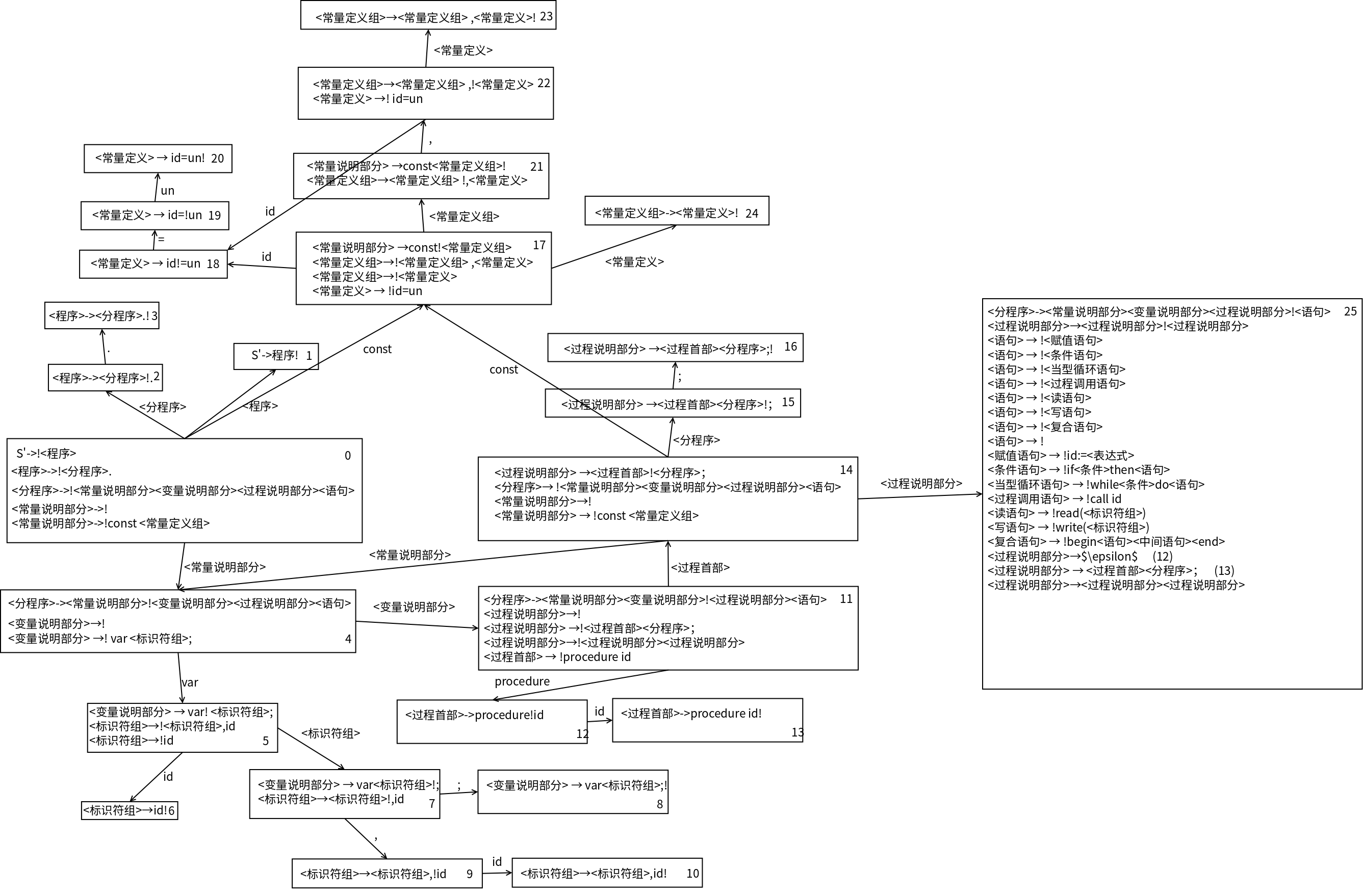于是决定开始写程序去算，具体的逻辑实在太过复杂，First，Follow还有最后的状态收集打印都花了我不少时间，这里直接讲述一下分析表生成程序的用法，所有关于分析表生成的程序都在状态表构造V2目录下：

3.1.将文法保存在gra.txt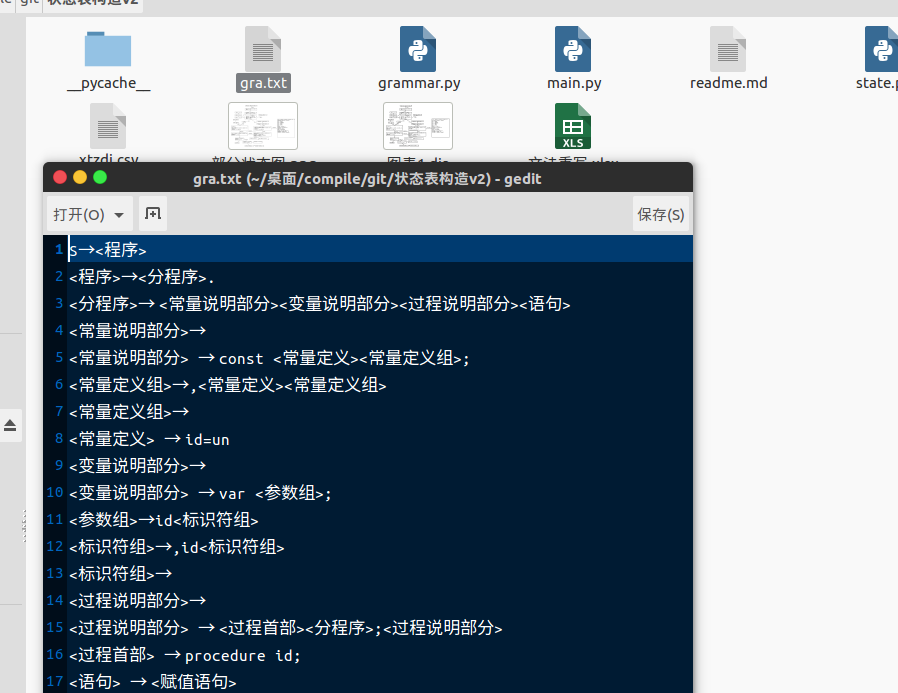如图，将自己的文法写到gra.txt中。

3.2.运行程序得到分析表

在命令行键入

python3 main.py

即可运行程序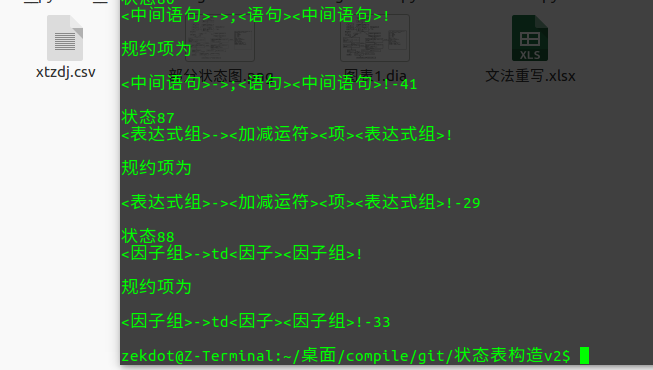如图，程序运行会打印出所有状态，可以利用管道把这个状态输出到文件中便于进行调试。这里的xtzdj.csv就是生成的分析表，可用Excel打开查看：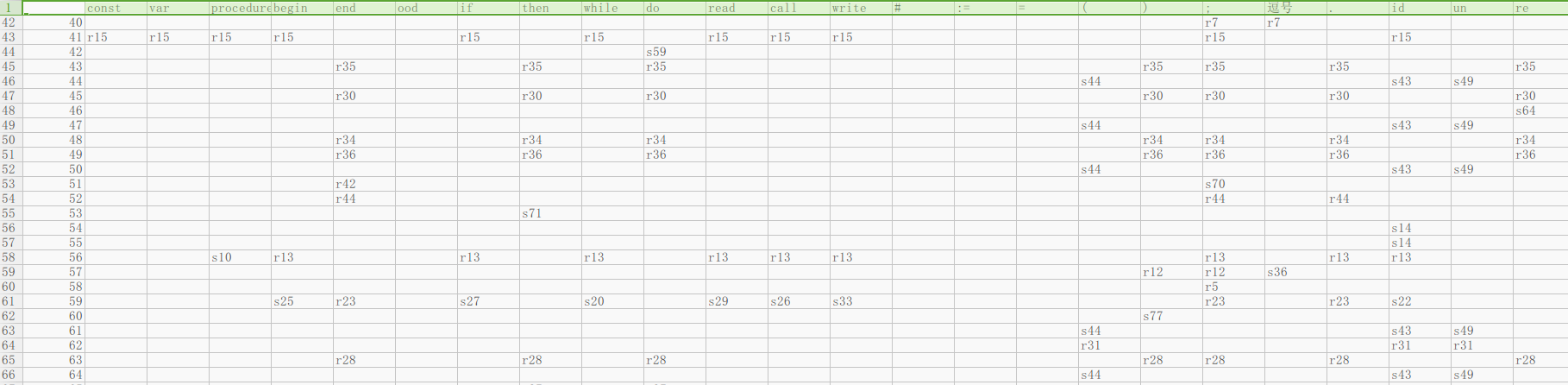这里行是状态，列是终结符或者非终结符，表中单元则是转移或者规约动作。

4.进行语法分析

得到语法分析表之后，就可以愉快的分析源程序的语法了，其实编写程序的过程并不愉快，文法的读取、状态的收集去重等还是花了我很长时间，这里也不具体讲述代码了，简单说一下程序执行方式：

4.1.把文法和语法分析表拷贝到语法分析树v2目录下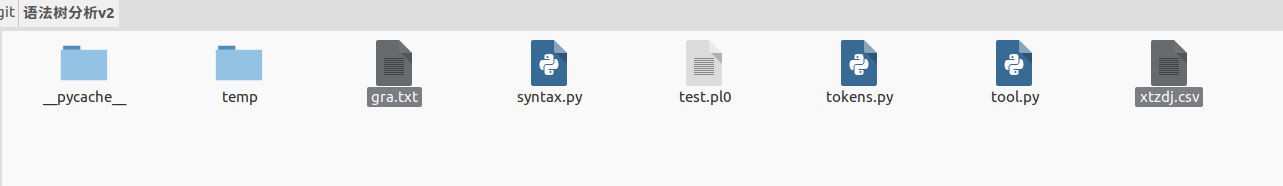如图，把这两个文件放到该目录下。

4.2.把要分析的源码写到目录下的test.pl0中

这里我的逻辑默认从test.pl0中读取源代码分析，如果需要的话可以修改syntax.py来修改这个默认名称。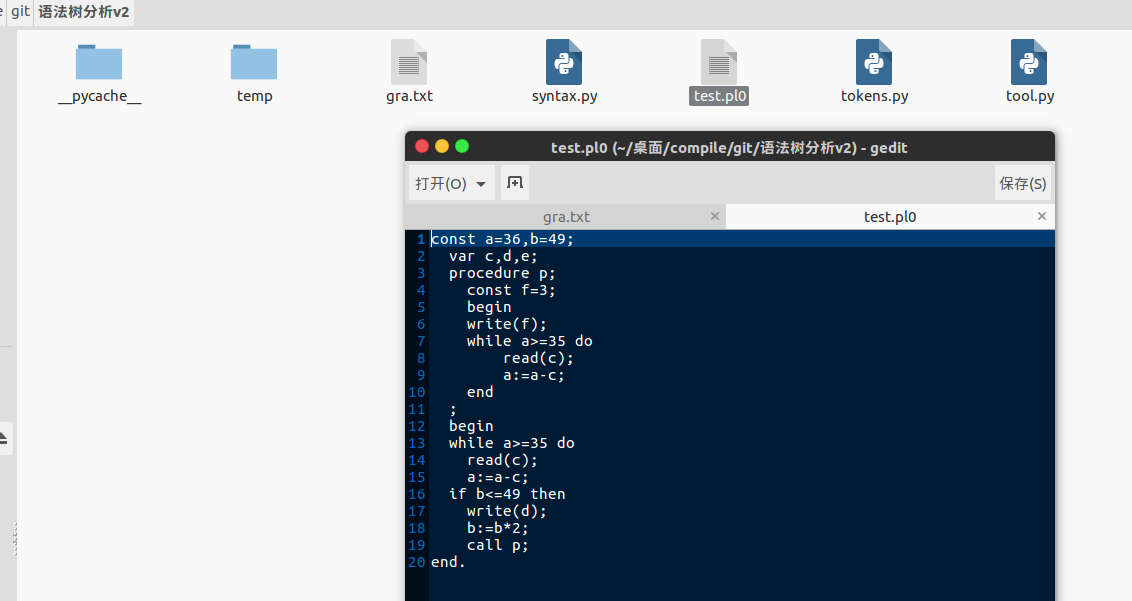4.3.运行语法分析程序

在命令行中键入

python3 syntax.py

即可运行程序。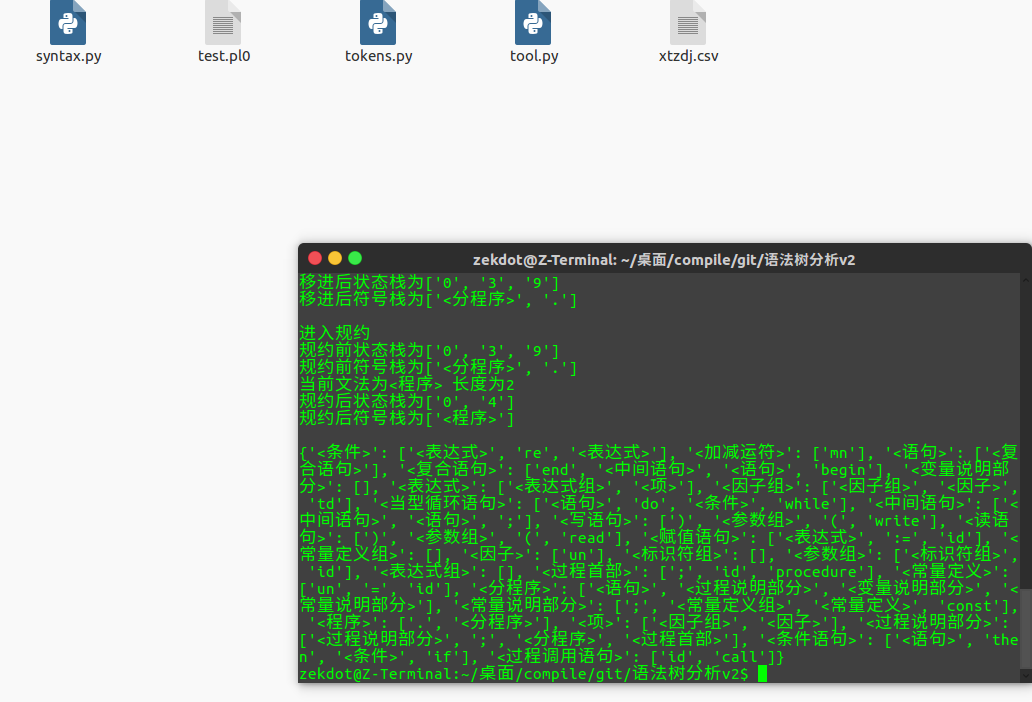如图所示，成功的识别了测试的pl0程序，并打印出了语法树的树枝。即语法分析成功。

其实到这一步整个编译器的工作并没有完成，接下来应该为其编写对应的属性文法，然后把源代码翻译成四元组，然后再是代码的优化，最后是执行，剩下的部分如果以后能闲下来再去探究吧。

展开全文编译原理 自底向上分析
• 自底向上语法分析相当于从叶子节点开始向上一直到根部构造一棵语法树。我们将使用移入-归约法完成这一过程。 归约 定义：一个与某产生式体相匹配的特定子串被替换成该产生式头部的非终结符号。相当于反向的最右...
• 自底向上分析概述 LR(0) 项目自动机 LR(0) 项 增广 构造自动机--项集，项集闭包，项集投影 内核项 与 非内核项 构造自动机--GOTO函数 移入规约冲突 SLR LR(1) LALR(1) LALR分析器与LR分析器 子问题 构造自动机--完整...编译原理
• 声明：本系列文章，是根据中国大学MOOC网 哈工大的编译原理 这门课学习而成的学习笔记。 一、自底向上分析概述 自底向上的语法分析 从分析树的底部(叶节点)...自底向上语法分析的通用框架 移入-归约分析(Shift-Re...
• 自底向上语法分析LR(1)

千次阅读 2019-10-17 13:42:45
自底向上分析方法，也称移进－归约分析法，粗略地说它的实现思想是对输入符号串左向右进行扫描，并将输入符逐个移入一个后进先出栈中，边移入边分析，一旦栈顶符号串形成某个句型的句柄时，就用该产生式的左部非...编译原理 自底向上 LR
• 这章和上一章顶向下是语法分析的两种方法。然而向上的分析方法更为强大一些，主要有: LR(0) SLR(1) LR(1) LALR(1) 我们仍然是以例子的方式，带大家慢慢了解这些知识点。 引用的课本: 《编译原理及实践》 ...编译原理 文法分析
• 编译器之语法分析自底向上基本概念算符优先SLR规范LRLALR 自底向上 基本概念 自底向上形成语法树的过程就是及逆行归约，将一堆单词串放在一起，形成某个产生式的样子，然后规约成某个产生式，所以关键就是什么时候...编译器
• 编译原理词法语法分析器 自底向上方法实现，会生成一个语法分析树
• 基于NFA(不确定有穷自动机)与自底向上语法分析构造的正则表达式解析
• LR语法分析器 自底向上分析的构造 包括文档和代码
• 编译原理-用例题理解-自底向上的语法分析 上一篇：编译原理-用例题理解-顶向下...自底向上语法分析从待输入的符号串开始，利用文法的产生式步步向上归约，试图归约到文法的开始符号。 从语法树的角度看 自底向上...
• 前言 最近的编译原理网课，真的是让人头大啊，尤其本校老师讲课风格有点照本宣科，ppt跳跃性和理论性都比较强，所以就导致这门课理解...LR(0)语法分析 最基础，也是最盲目的分析方法。 分析的步骤大概是： 构造状...经验分享
• 编译原理作业，递归下降语法分析器。根据课堂讲授的形式化算法，编制程序实现递归下降分析器，能对常见的语句进行分析。
• 该程序可以直接在Visual C++ 6.0下直接运行，但是必须保证该工程下有一个sentence.txt的文本文件保存待分析的句子
• 对文法进行自动分析，生成用于SLR1语法分析器的状态转换表，加上框架代码，构造出SLR1语法分析程序
• 系列文章目录 【学习笔记】编译原理——第一章 编译引论 【学习笔记】编译原理——第二章 词法分析 【学习笔记】编译原理——第四章 自上而下分析法 ...自底向上语法分析的通用框架——移入归约分析 栈的左侧为栈
• 第五章 自底向上语法分析  重点：自底向上分析的基本思想，算符优先分析法的基本思想，简单算符优先分析法。LR分析器的基本构造思想，LR分析算法，规范句型活前缀及其识别器——DFA，LR(0)分析表的构造，SLR...
• 先用代码占个坑，等期末结束了再补详细解释。。。 /* 2020-06-20 by 软件工程172 202171139 TDM-GCC 4.9.2 64-bit -std=C++11 */ #include #... cout 算符优先分析表\n4.检测输入串\n5.退出\n"; while(true) { cout...
• LR语法分析器

2021-05-22 06:27:01
1LR语法分析器本节介绍一个有效的自底向上的分析技术，可以用于一大类上下文无关文法的语法分析。这种技术叫做LR(k)分析法，其中L表示从左到右扫描输入串，R表示构造一个最右推导的逆过程，k指的是在决定语法分析...
• 目录自底向上优先分析概述**简单优先分析法算符优先分析法算符优先分析法概述直观算符优先分析法算符优先文法的定义算符优先关系表的构造算符优先分析算法算符优先分析法的局限LR 分析LR 分析器概述 自底向上优先...
• 翻译的另外两个方法：递归（构造递归程序）、自底向上构造（有实例）。分析栈 词法分析器...